# Lecture 2

## Compiling

• Last time, we learned to write our first program in C, printing “hello, world” to the screen.
• We compiled it with make hello first, turning our source code into machine code before we could run the compiled program with ./hello.
• make is actually just a program that calls clang, a compiler, with options. We could compile our source code file, hello.c, ourselves by running the command clang hello.c. Nothing seems to happen, which means there were no errors. And if we run ls, now we see an a.out file in our directory. The filename is still the default, so we can actually run a more specific command: clang -o hello hello.c.
• We’ve added another command-line argument, or an input to a program on the command-line as extra words after the program’s name. clang is the name of the program, and -o, hello, and hello.c are additional arguments. We’re telling clang to use hello as the output filename, and use hello.c as the source code. Now, we can see hello being created as output.
• If we wanted to use CS50’s library, via #include <cs50.h>, for the get_string function, we also have to add a flag: clang -o hello hello.c -lcs50:
#include <cs50.h>
#include <stdio.h>

int main(void)
{
string name = get_string("What's your name? ");
printf("hello, %s\n", name);
}

• The -l flag links the cs50 file, which is already installed in the CS50 IDE, and includes the machine code for get_string (among other functions) that our program can then refer to and use as well.
• With make, these arguments are generated for us since the staff has configured make in the CS50 IDE already as well.
• Compiling source code into machine code is actually made up of smaller steps:
• preprocessing
• compiling
• assembling
• Preprocessing generally involves lines that start with a #, like #include. For example, #include <cs50.h> will tell clang to look for that header file, since it contains content that we want to include in our program. Then, clang will essentially replace the contents of those header files into our program.
• For example …
#include <cs50.h>
#include <stdio.h>

int main(void)
{
string name = get_string("What's your name? ");
printf("hello, %s\n", name);
}

• … will be preprocessed into:
...
string get_string(string prompt);
int printf(string format, ...);
...

int main(void)
{
string name = get_string("Name: ");
printf("hello, %s\n", name);
}

• This includes the prototypes of all the functions from those libraries we included, so we can then use them in our code.
• Compiling takes our source code, in C, and converts it to another type of source code called assembly code, which looks like this:
...
main:                         # @main
.cfi_startproc
# BB#0:
pushq    %rbp
.Ltmp0:
.cfi_def_cfa_offset 16
.Ltmp1:
.cfi_offset %rbp, -16
movq    %rsp, %rbp
.Ltmp2:
.cfi_def_cfa_register %rbp
subq    $16, %rsp xorl %eax, %eax movl %eax, %edi movabsq$.L.str, %rsi
movb    $0, %al callq get_string movabsq$.L.str.1, %rdi
movq    %rax, -8(%rbp)
movq    -8(%rbp), %rsi
movb    \$0, %al
callq    printf
...

• These instructions are lower-level and is closer to the binary instructions that a computer’s processor can directly understand. They generally operate on bytes themselves, as opposed to abstractions like variable names.
• The next step is to take the assembly code and translate it to instructions in binary by assembling it. The instructions in binary are called machine code, which a computer’s CPU can run directly.
• The last step is linking, where previously compiled versions of libraries that we included earlier, like cs50.c, are actually combined with the binary of our program. So we end up with one binary file, a.out or hello, that is the combined machine code for hello.c, cs50.c, and stdio.c. (In the CS50 IDE, precompiled machine code for cs50.c and stdio.c has already been installed, and clang has been configured to find and use them.)
• These four steps have been abstracted away, or simplified, by make, so all we have to implement is the code for our programs.

## Debugging

• Bugs are mistakes or problems in programs that cause them to behave differently than intended. And debugging is the process of finding and fixing those bugs.
• Last week, we learned about a few tools that help us with writing code that compiles, has good style, and is correct:
• help50
• style50
• check50
• We can use another “tool”, the printf function, to print messages and variables to help us debug.
• Let’s take a look at buggy0.c:
#include <stdio.h>

int main(void)
{
// Print 10 hashes
for (int i = 0; i <= 10; i++)
{
printf("#\n");
}
}

• Hmm, we want to print only 10 #s, but there are 11. If we didn’t know what the problem is (since our program is compiling without any errors, and we now have a logical error), we could add another printf temporarily:
#include <stdio.h>

int main(void)
{
for (int i = 0; i <= 10; i++)
{
printf("i is now %i\n", i);
printf("#\n");
}
}

• Now, we can see that i started at 0 and continued until it was 10, but we should have our for loop stop once it’s at 10, with i < 10 instead of i <= 10.
• In the CS50 IDE, we have another tool, debug50, to help us debug programs. This is a tool written by staff that’s built on a standard tool called gdb. Both of these debuggers are programs that will run our own programs step-by-step and let us look at variables and other information while our program is running.
• We’ll run the command debug50 ./buggy0, and it will tell us to recompile our program since we changed it. Then, it’ll tell us to add a breakpoint, or indicator for a line of code where the debugger should pause our program.
• By using the up and down keys in the terminal, we can reuse commands from the past without typing them again.
• We’ll click to the left of line 6 in our code, and a red circle will appear: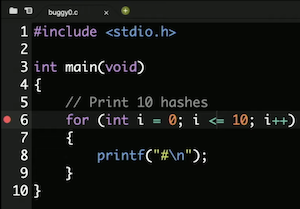• Now, if we run debug50 ./buggy0 again, we’ll see the debugger panel open on the right: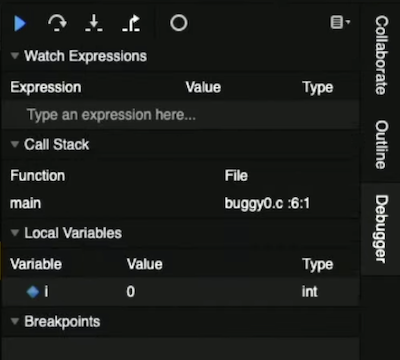• We see that the variable we made, i, is under the Local Variables section, and see that there’s a value of 0.
• Our breakpoint has paused our program on line 6, highlighting that line in yellow. To continue, we have a few controls in the debugger panel. The blue triangle will continue our program until we reach another breakpoint or the end of our program. The curved arrow to its right, Step Over, will “step over” the line, running it and pausing our program again immediately after.
• So, we’ll use the curved arrow to run the next line, and see what changes after. We’re at the printf line, and pressing the curved arrow again, we see a single # printed to our terminal window. With another click of the arrow, we see the value of i change to 1. We can keep clicking the arrow to watch our program run, one line at a time.
• To exit the debugger, we can press control + C to stop the running program.
• Let’s look at another example, buggy1.c:
#include <cs50.h>
#include <stdio.h>

// Prototype
int get_negative_int(void);

int main(void)
{
// Get negative integer from user
int i = get_negative_int();
printf("%i\n", i);
}

int get_negative_int(void)
{
int n;
do
{
n = get_int("Negative Integer: ");
}
while (n < 0);
return n;
}

• We’ve implemented another function, get_negative_int, to get a negative integer from the user. We need to remember the prototype before our main function, and then our code compiles.
• But when we run our program, it keeps asking us for a negative integer, even after we provide one. We’ll set a breakpoint on line 10, int i = get_negative_int();, since it’s the first interesting line of code. We’ll run debug50 ./buggy1, and see in the debugging panel’s Call Stack section that we’re in the main function. (The “call stack” refers to all the functions that have been called in our program at the time, and not yet returned from. So far, only the main function has been called.)
• We’ll click the arrow pointing down, Step Into, and the debugger brings us into the function called on that line, get_negative_int. We see the call stack updated with the function’s name, and the variable n with a value of 0: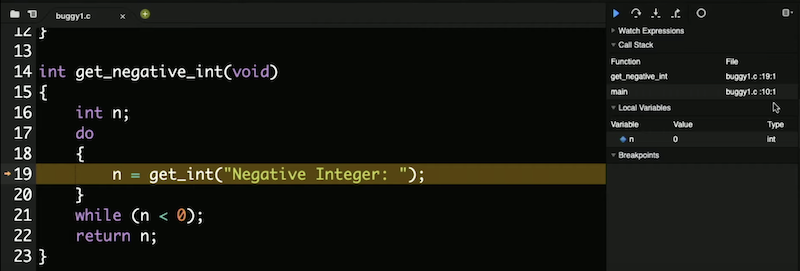• We can click the Step Over arrow again, and see n be updated with -1, which is indeed what we entered: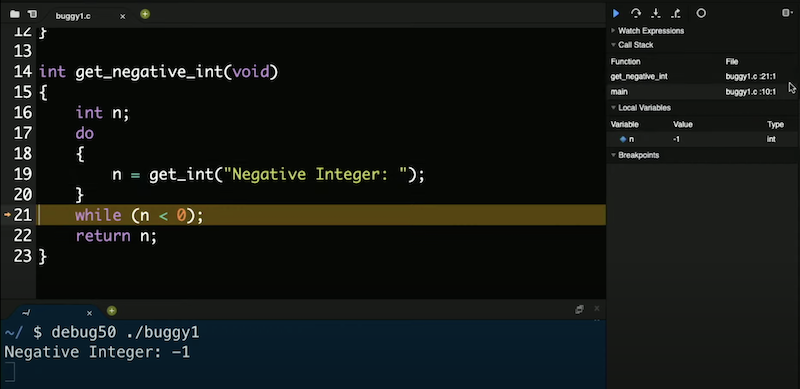• We click Step Over again, and we see our program going back inside the loop. Our while loop is still running, so the condition that it checks must be true still. And we do see that n < 0 is true even if we entered a negative integer, so we should fix our bug by changing it to n >= 0.
• We can save lots of time in the future by investing a little bit now to learn how to use debug50!
• We can also use ddb, short for “duck debugger”, a real technique where we explain what we’re trying to do to a rubber duck, and oftentimes we’ll realize our own mistake in logic or implementation as we’re explaining it.

## Memory

• In C, we have different types of variables we can use for storing data, and each of them take up a fixed amount of space. Different computer systems actually vary in the amount of space actually used for each type, but we’ll work with the amounts here, as used in the CS50 IDE:
• bool 1 byte
• char 1 byte
• double 8 bytes
• float 4 bytes
• int 4 bytes
• long 8 bytes
• string ? bytes
• Inside our computers, we have chips called RAM, random-access memory, that stores data for short-term use, like a program’s code while it’s running, or a file while it’s open. We might save a program or file to our hard drive (or SSD, solid state drive) for long-term storage, but use RAM because it is much faster. However, RAM is volatile, or requires power to keep data stored.
• We can think of bytes stored in RAM as though they were in a grid: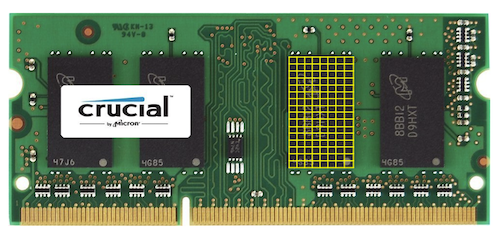• In reality, there are millions or billions of bytes per chip.
• Each byte will have a location on the chip, like the first byte, second byte, and so on.
• In C, when we create a variable of type char, which will be sized one byte, it will physically be stored in one of those boxes in RAM. An integer, with 4 bytes, will take up four of those boxes.

## Arrays

• Let’s say we wanted to take the average of three variables:
#include <stdio.h>

int main(void)
{
int score1 = 72;
int score2 = 73;
int score3 = 33;

printf("Average: %f\n", (score1 + score2 + score3) / 3.0);
}

• We divide by not 3, but 3.0 so the result is also a float.
• We can compile and run our program, and see an average printed.
• While our program is running, the three int variables are stored in memory: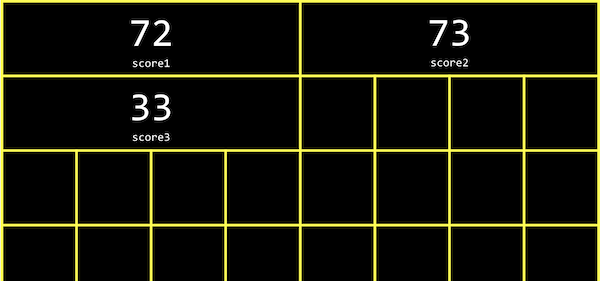• Each int takes up four boxes, representing four bytes, and each byte in turn is made up of eight bits, 0s and 1s stored by electrical components.
• It turns out, in memory, we can store variables one after another, back-to-back, and access them more easily with loops. In C, a list of values stored one after another contiguously is called an array.
• For our program above, we can use int scores; to declare an array of three integers instead.
• And we can assign and use variables in an array with scores = 72. With the brackets, we’re indexing into, or going to, the “0th” position in the array. Arrays are zero-indexed, meaning that the first value has index 0, and the second value has index 1, and so on.
• Let’s update our program to use an array:
#include <cs50.h>
#include <stdio.h>

int main(void)
{
int scores;
scores = get_int("Score: ");
scores = get_int("Score: ");
scores = get_int("Score: ");

// Print average
printf("Average: %f\n", (scores + scores + scores) / 3.0);
}

• Now, we’re asking the user for three values, and printing the average as before, but using the values stored in the array.
• Since we can set and access items in an array based on their position, and that position can also be the value of some variable, we can use a loop:
#include <cs50.h>
#include <stdio.h>

int main(void)
{
int scores;
for (int i = 0; i < 3; i++)
{
scores[i] = get_int("Score: ");
}

// Print average
printf("Average: %f\n", (scores + scores + scores) / 3.0);
}

• Now, instead of hard-coding, or manually specifying each element three times, we use a for loop and i as the index of each element in the array.
• And we repeated the value 3, representing the length of our array, in two different places. So we can use a constant, or variable with a fixed value in our program:
#include <cs50.h>
#include <stdio.h>

const int TOTAL = 3;

int main(void)
{
int scores[TOTAL];
for (int i = 0; i < TOTAL; i++)
{
scores[i] = get_int("Score: ");
}

printf("Average: %f\n", (scores + scores + scores) / TOTAL);
}

• We can use the const keyword to tell the compiler that the value of TOTAL should never be changed by our program. And by convention, we’ll place our declaration of the variable outside of the main function and capitalize its name, which isn’t necessary for the compiler but shows other humans that this variable is a constant and makes it easy to see from the start.
• But now our average will be incorrect or broken if we don’t have exactly three values.
• Let’s add a function to calculate the average:
float average(int length, int array[])
{
int sum = 0;
for (int i = 0; i < length; i++)
{
sum += array[i];
}
return sum / (float) length;
}

• We’ll pass in the length and an array of ints (which could be any size), and use another loop inside our helper function to add up the values into a sum variable. We use (float) to cast length into a float, so the result we get from dividing the two is also a float.
• Now, in our main function, we can call our new average function with printf("Average: %f\n", average(TOTAL, scores);. Notice that the names of the variables in main don’t need to match what average calls them, since only the values are passed in.
• We need to pass in the length of the array to the average function, so it knows how many values there are.

## Characters

• We can print out a single character with a simple program:
#include <stdio.h>

int main(void)
{
char c = '#';

printf("%c\n", c);
}

• When we run this program, we get # printed in the terminal.
• Let’s see what happens if we change our program to print c as an integer:
#include <stdio.h>

int main(void)
{
char c = '#';

printf("%i\n", (int) c);
}

• When we run this program, we get 35 printed. It turns out that 35 is indeed the ASCII code for a # symbol.
• In fact, we don’t need to cast c to an int explicitly; the compiler can do that for us in this case.
• A char is a single byte, so we can picture it as being stored in one box in the grid of memory above.

## Strings

• We can print out a string, or some text, by creating a variable for each character and printing them out:
#include <stdio.h>

int main(void)
{
char c1 = 'H';
char c2 = 'I';
char c3 = '!';

printf("%c%c%c\n", c1, c2, c3);
}

• Here, we’ll see HI! printed out.
• Now let’s print out the integer values of each character:
#include <stdio.h>

int main(void)
{
char c1 = 'H';
char c2 = 'I';
char c3 = '!';

printf("%i %i %i\n", c1, c2, c3);
}

• We’ll see 72 73 33 printed out, and realize that these characters are stored in memory like so: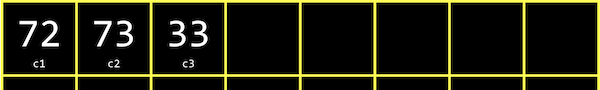• Strings are actually just arrays of characters, and defined not in C but by the CS50 library. If we had an array called s, each character can be accessed with s, s, and so on.
• And it turns out that a string ends with a special character, '\0', or a byte with all bits set to 0. This character is called the null character, or NUL. So we actually need four bytes to store our string with three characters: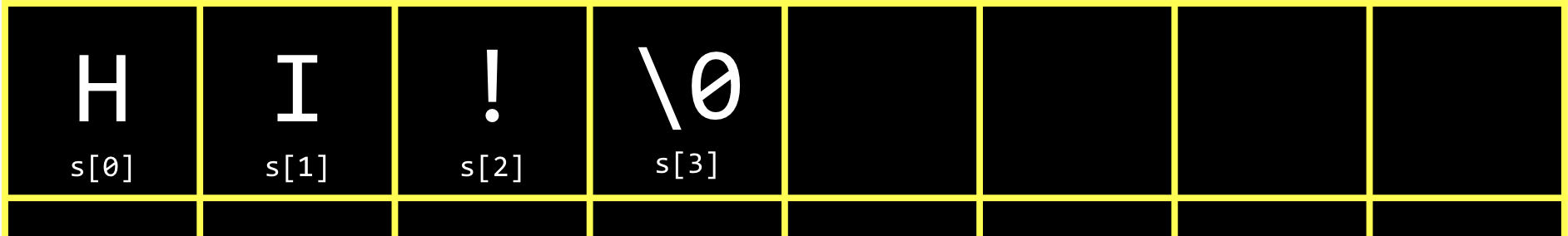• We can use a string as an array in our program, and print out the ASCII codes, or integer values, of each character in the string:
#include <cs50.h>
#include <stdio.h>

int main(void)
{
string s = "HI!";
printf("%i %i %i %i\n", s, s, s, s);
}

• And as we might have expected, we see 72 73 33 0 printed.
• In fact, we could try to access s, and see some unexpected symbol printed. With C, our code has the ability to access or change memory that it otherwise shouldn’t, which is both powerful and dangerous.
• We can use a loop to print out every character in a string:
#include <cs50.h>
#include <stdio.h>

int main(void)
{
string s = get_string("Input:  ");
printf("Output: ");
for (int i = 0; s[i] != '\0'; i++)
{
printf("%c", s[i]);
}
printf("\n");
}

• We can change our loop’s condition to continue regardless of what i is, but rather only when s[i] != '\0', or when the character at the current position in s isn’t the null character.
• We can use a function that comes with C’s string library, strlen, to get the length of the string for our loop:
#include <cs50.h>
#include <stdio.h>
#include <string.h>

int main(void)
{
string s = get_string("Input:  ");
printf("Output: ");
for (int i = 0; i < strlen(s); i++)
{
printf("%c", s[i]);
}
printf("\n");
}

• We have an opportunity to improve the design of our program. Our loop was a little inefficient, since we check the length of the string, after each character is printed, in our condition. But since the length of the string doesn’t change, we can check the length of the string once:
#include <cs50.h>
#include <stdio.h>
#include <string.h>

int main(void)
{
string s = get_string("Input: ");
printf("Output:\n");
for (int i = 0, n = strlen(s); i < n; i++)
{
printf("%c\n", s[i]);
}
}

• Now, at the start of our loop, we initialize both an i and n variable, and remember the length of our string in n. Then, we can check the values without having to call strlen to calculate the length of the string each time.
• And we did need to use a little more memory to store n, but this saves us some time with not having to check the length of the string each time.
• We might declare an array of two strings:
string words;
words = "HI!";
words = "BYE!";

• And in memory, the array of strings might be stored and accessed with:• words refers to the first element, or value, of the words array, which is a string, and so words refers to the first element in that string, which is a character.
• So an array of strings is just an array of arrays of characters.
• We can now combine what we’ve seen, to write a program that can capitalize letters:
#include <cs50.h>
#include <stdio.h>
#include <string.h>

int main(void)
{
string s = get_string("Before: ");
printf("After:  ");
for (int i = 0, n = strlen(s); i < n; i++)
{
if (s[i] >= 'a' && s[i] <= 'z')
{
printf("%c", s[i] - 32);
}
else
{
printf("%c", s[i]);
}
}
printf("\n");
}

• First, we get a string s from the user. Then, for each character in the string, if it’s lowercase (which means it has a value between that of a and z), we convert it to uppercase. Otherwise, we just print it.
• We can convert a lowercase letter to its uppercase equivalent by subtracting the difference between their ASCII values. (We know that lowercase letters have a higher ASCII value than uppercase letters, and the difference is the same between the same letters, so we can subtract to get an uppercase letter from a lowercase letter.)
• It turns out that there’s another library, ctype.h, that we can use:
#include <cs50.h>
#include <ctype.h>
#include <stdio.h>
#include <string.h>

int main(void)
{
string s = get_string("Before: ");
printf("After:  ");
for (int i = 0, n = strlen(s); i < n; i++)
{
if (islower(s[i]))
{
printf("%c", toupper(s[i]));
}
else
{
printf("%c", s[i]);
}
}
printf("\n");
}

• Now, our code is more readable and likely to be correct, since others have written and tested these functions for us.
• We can simplify even further, and just pass in each character to toupper, since it doesn’t change non-lowercase characters:
#include <cs50.h>
#include <ctype.h>
#include <stdio.h>
#include <string.h>

int main(void)
{
string s = get_string("Before: ");
printf("After:  ");
for (int i = 0, n = strlen(s); i < n; i++)
{
printf("%c", toupper(s[i]));
}
printf("\n");
}

• We can use CS50’s manual pages to find and learn about common library functions. From searching the man pages, we see toupper() is a function, among others, from a library called ctype, that we can use.

## Command-line arguments

• Programs of our own can also take in command-line arguments, or words added after our program’s name in the command itself.
• In argv.c, we change what our main function looks like:
#include <cs50.h>
#include <stdio.h>

int main(int argc, string argv[])
{
if (argc == 2)
{
printf("hello, %s\n", argv);
}
else
{
printf("hello, world\n");
}
}

• argc and argv are two variables that our main function will now get automatically when our program is run from the command line. argc is the argument count, or number of arguments, and argv, argument vector (or argument list), an array of strings.
• The first argument, argv, is the name of our program (the first word typed, like ./hello). In this example, we check if we have two arguments, and print out the second one if so.
• For example, if we run ./argv David, we’ll get hello, David printed, since we typed in David as the second word in our command.
• We can print out each character individually, too:
#include <cs50.h>
#include <stdio.h>
#include <string.h>

int main(int argc, string argv[])
{
if (argc == 2)
{
for (int i = 0, n = strlen(argv); i < n; i++)
{
printf("%c\n", argv[i]);
}
}
}

• We’ll use argv[i] to access each character in the first argument to our program.
• It turns out that our main function also returns an integer value. By default, our main function returns 0 to indicate nothing went wrong, but we can write a program to return a different value:
#include <cs50.h>
#include <stdio.h>

int main(int argc, string argv[])
{
if (argc != 2)
{
printf("missing command-line argument\n");
return 1;
}
printf("hello, %s\n", argv);
return 0;
}

• The return value of main in our program is called an exit code, usually used to indicate error codes. (We’ll write return 0 explicitly at the end of our program here, even though we don’t technically need to.)
• As we write more complex programs, error codes like this can help us determine what went wrong, even if it’s not visible or meaningful to the user

## Applications

• Now that we know how to work with strings in our programs, as well code written by others in libraries, we can analyze paragraphs of text for their level of readability, based on factors like how long and complicated the words and sentences are.
• Cryptography is the art of scrambling, or hiding information. If we wanted to send a message to someone, we might want to encrypt, or somehow scramble that message so that it would be hard for others to read. The original message, or input to our algorithm, is called plaintext, and the encrypted message, or output, is called ciphertext. And the algorithm that does the scrambling is called a cipher. A cipher generally requires another input in addition to the plaintext. A key, like a number, is some other input that is kept secret.
• For example, if we wanted to send a message like I L O V E Y O U, we can first convert it to ASCII: 73 76 79 86 69 89 79 85. Then, we can encrypt it with a key of just 1 and a simple algorithm, where we just add the key to each value: 74 77 80 87 70 90 80 86. Then, the ciphertext after we convert the values back to ASCII would be J M P W F Z P V. To decrypt this, someone would have to know the key is 1, and to subtract it from each character!
• We’ll apply these concepts in our sections and problem set!